# AP Statistics : How to use the Central Limit Theorem

## Example Questions

### Example Question #1 : How To Use The Central Limit Theorem

Let us suppose we have population data where the data are distributed Poisson

(see the figure for an example of a Poisson random variable).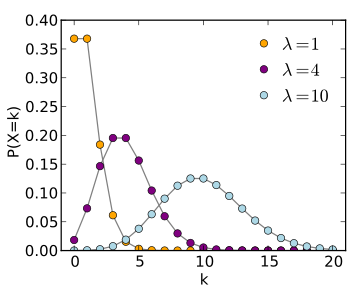Which distribution increasingly approximates the sample mean as the sample size increases to infinity?

Gamma

Exponential

Normal

Poisson

Normal

Explanation:

The Central Limit Theorem holds that for any distribution with finite mean and variance the sample mean will converge in distribution to the normal as sample size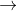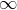.

### Example Question #2 : How To Use The Central Limit Theorem

Cat owners spend an average of $40 per month on their pets, with a standard deviation of$5.

What is the probability that a randomly selected pet owner spends less than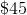a month on their pet?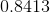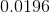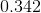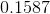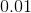Explanation:

First, draw the distribution and the area you are interested in.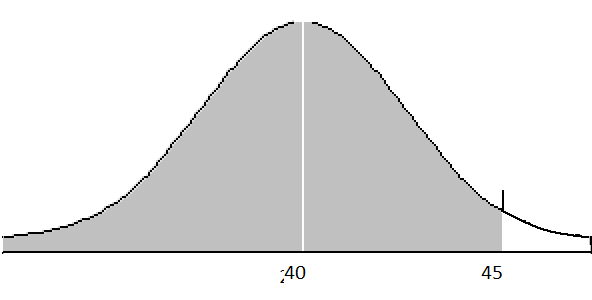Next, calculate the z-score for the person of interest. Because the population standard deviation is known, we will use the formula for z-score and not t-score.

We will find the z-score for the person of interest, and then calculate the area under the curve that falls below, or to the left of that z-score.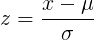Now we will find the value for each variable given in the problem: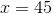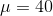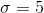Third, solve for z using the information in the problem.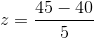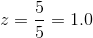Now we must determine the area under the curve to the left of a z-score of 1.0. We will consult a z-table.

Look up 1.0 in the row and 0.00 in the column.

If your z-table gives shaded area to the left, you will get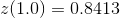We are interested in area to the left, which is what we found, so this is our answer.

If your z-table gives shaded area to the right, you will get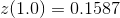Because we want the area to the left of z=1.0, we will subtract that area from 1: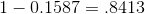### Example Question #3 : How To Use The Central Limit Theorem

It is known that cat owners spend an average of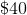a month on their pets, with a standard deviation of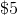.

What is the probability that a randomly selected cat owner spends between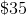toa month on their pet?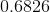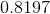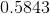Explanation:

First, draw the distribution and the area you are interested in.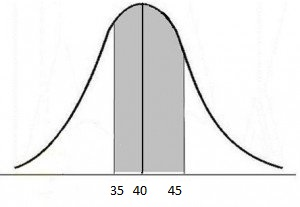Next, we will need to calculate z-scores for both values we are interested in, and find the area under the curve that falls between these two values.

Because the population standard deviation is known, we will use the formula for z-score and not t-score.We will find the z-score for the lower bound and find the z-score for the upper bound.

For the lower bound:Now we will do the same for the upper bound: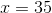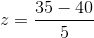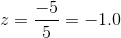Now we must determine the area under the curve which falls between z-scores of -1.0 and 1.0. To do this we will look up both z-scores and then subtract their areas (subtract the smaller area from the bigger area, so that you don't get a negative value).

For the lower bound, look up 1.0 in the row and 0.00 in the column. Then, for the upper bound, look up -1.0 in the row and 0.00 in the column.

The area to the left of 1.0 is 0.8413.

The area to the left of -1.0 is 0.1587.

To find the area between the two z-scores just subtract: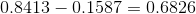The central limit theorem states that the area under the curve + or - 1 standard deviation from the mean is 68.3%, which we have confirmed.

### Example Question #4 : How To Use The Central Limit Theorem

Cat owners spend an average ofper month on their pets, with a standard deviation of.

What is the probability that a randomly chosen cat owner spends more thana month on their pet?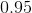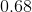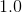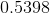Explanation:

First, draw the appropriate curve to represent the problem: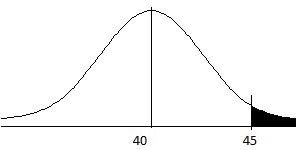Because we want greater than 45, shade the right hand portion of the graph.

We will be using the z-distribution and not the t-distribution because we know the population standard deviation.

We will find the z-score for this cat owner, and then find the area to the right of that z-score to find the probability of spending more than \$45.

We begin with the z-score formula: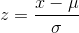Now find each value from the information given:Fill in the formula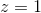Now look this z-score up in the z-table to find the area under the curve. Look up 1.0 in the row and 0.00 in the column.

If your z table gives area shaded to the left, you will findYou want the area to the right, so subtract the above from 1.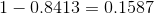If your z table gives area shaded to the right, you will findBecause you want the area to the right of z=1.0, this is your answer.

### Example Question #5 : How To Use The Central Limit Theorem

If the Central Limit Theorem applies, we can infer that:

The sampling distribution will be approximately normally distributed.

The standard deviation will be small.

The sample mean will be close to the population mean.

Every possible sample mean will be equal to the population mean.

The sampling distribution will be approximately normally distributed.

Explanation:

The Central Limit Theorem tells us that when the theorem applies, the sampling distribution will be approximately normally distributed.

### Example Question #6 : How To Use The Central Limit Theorem

A survey company samples 60 randomly selected college students to see if they own an American Express credit card. One percent of all college students own an American Express credit card. Does the Central Limit Theorem apply?

No.

Not enough information is given.

Yes.

No.

Explanation:

No. Whenever we get a "proportion" question we need to check whether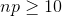and whether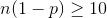.

In this problem,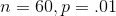.

Therefore,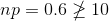.

So the central limit theorem does not apply.

### Example Question #7 : How To Use The Central Limit Theorem

In a particular library, there is a sign in the elevator that indicates a limit of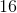persons  and a weight limit of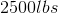. Assume an approximately normal distribution, that the average weight of students, faculty, and staff on campus is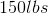, and the standard deviation is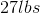.

If a random sample of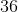people is taken, what is the standard deviation of their weights?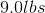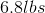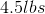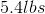This question deals with the Central Limit Theorem, which states that a random sample taken from a large population where the sampling distribution of sample averages is approximately normal has a standard deviation equal to the standard deviation of the population divided by the square root of the sample size. The information given allows us to apply the Central Limit Theorem as it satisfies the necessary characteristics of the sampling distribution/size. The standard deviation of the population is 27lbs, and the sample size is 36; therefore, the standard deviation of the 36-person random sample is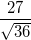, which gives us 4.5lbs.